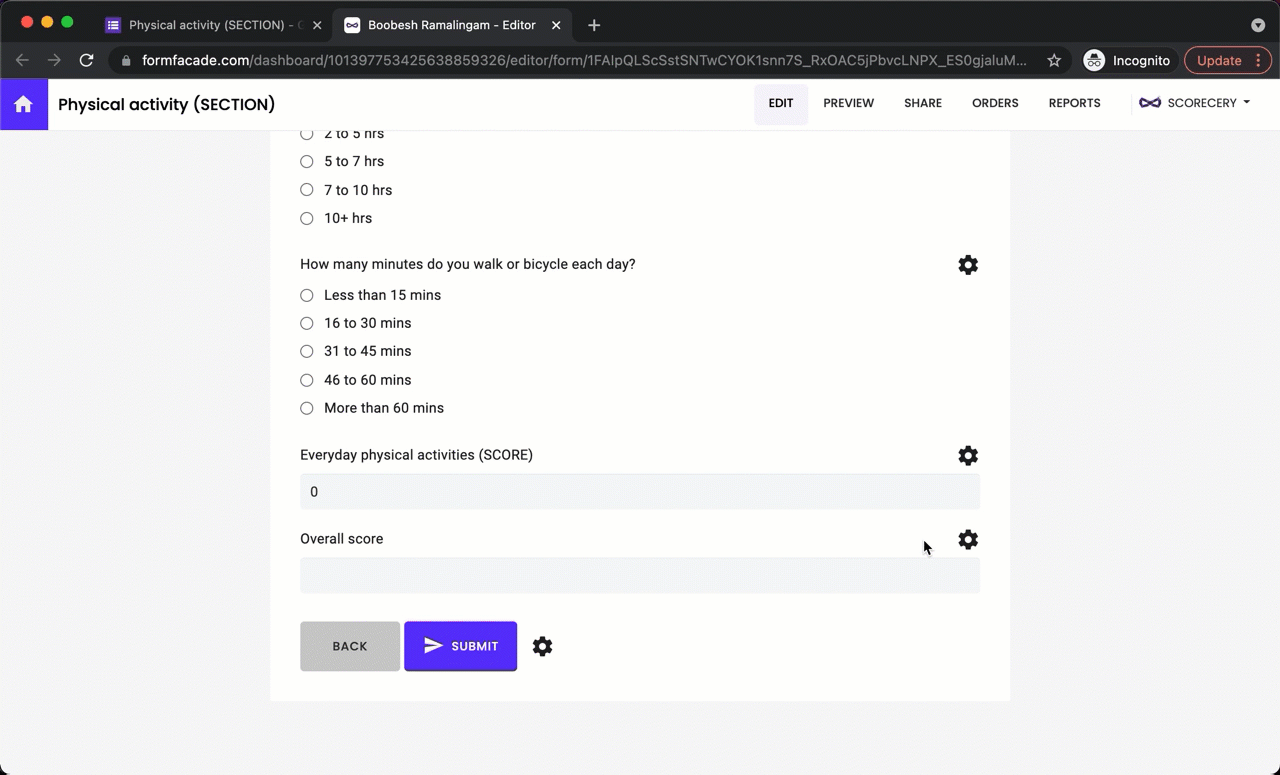# How to calculate scores for each section in Google Forms?

### You can assign point values to questions in Google Forms by creating a quiz and calculate the total score, but you cannot calculate the individual scores for each section. You can use the Scorecery addon for score calculations.

#### How to calculate individual scores for each section?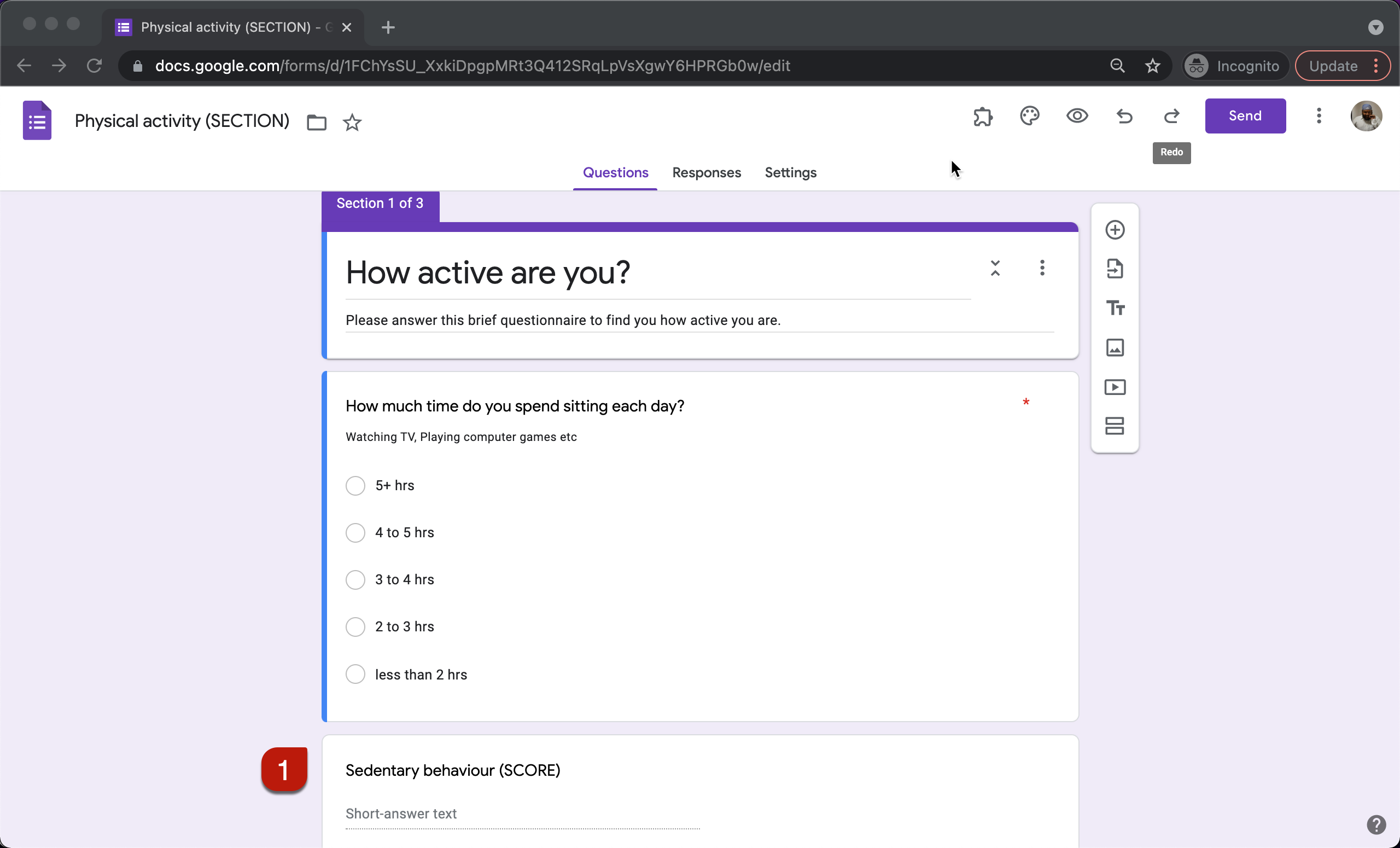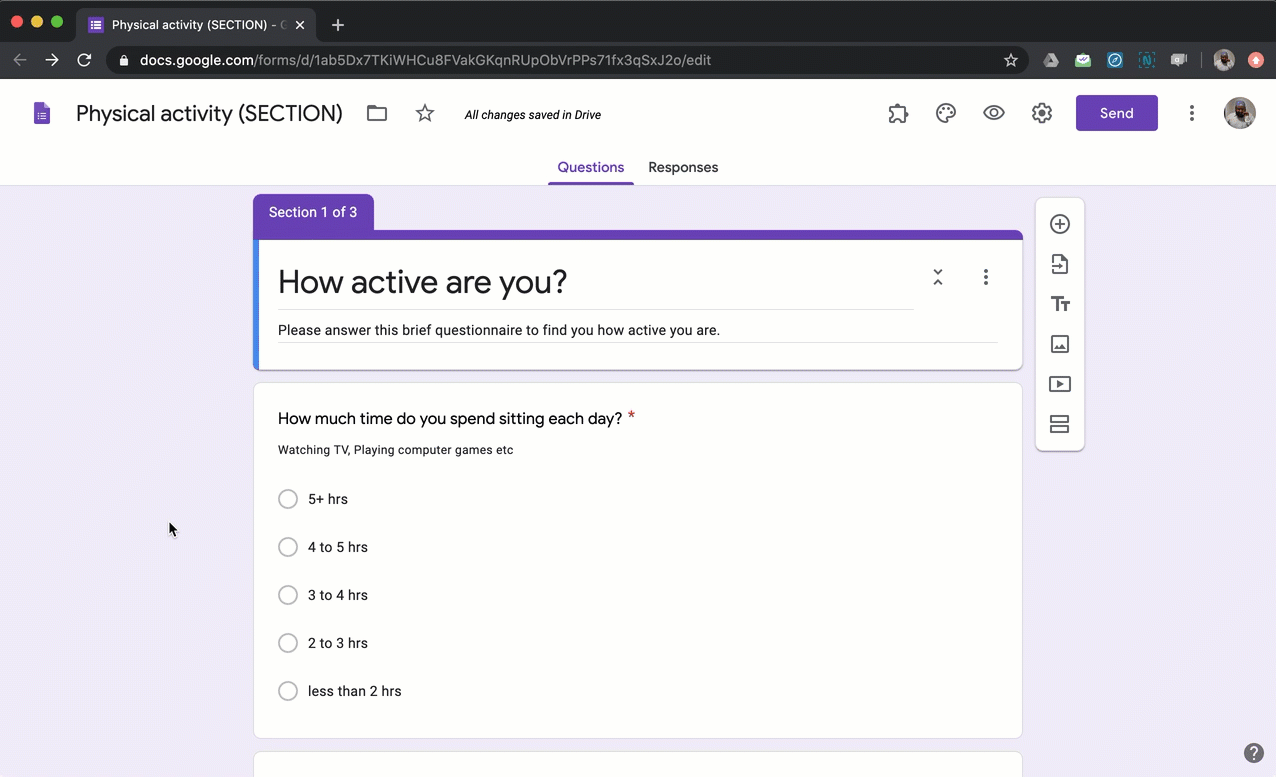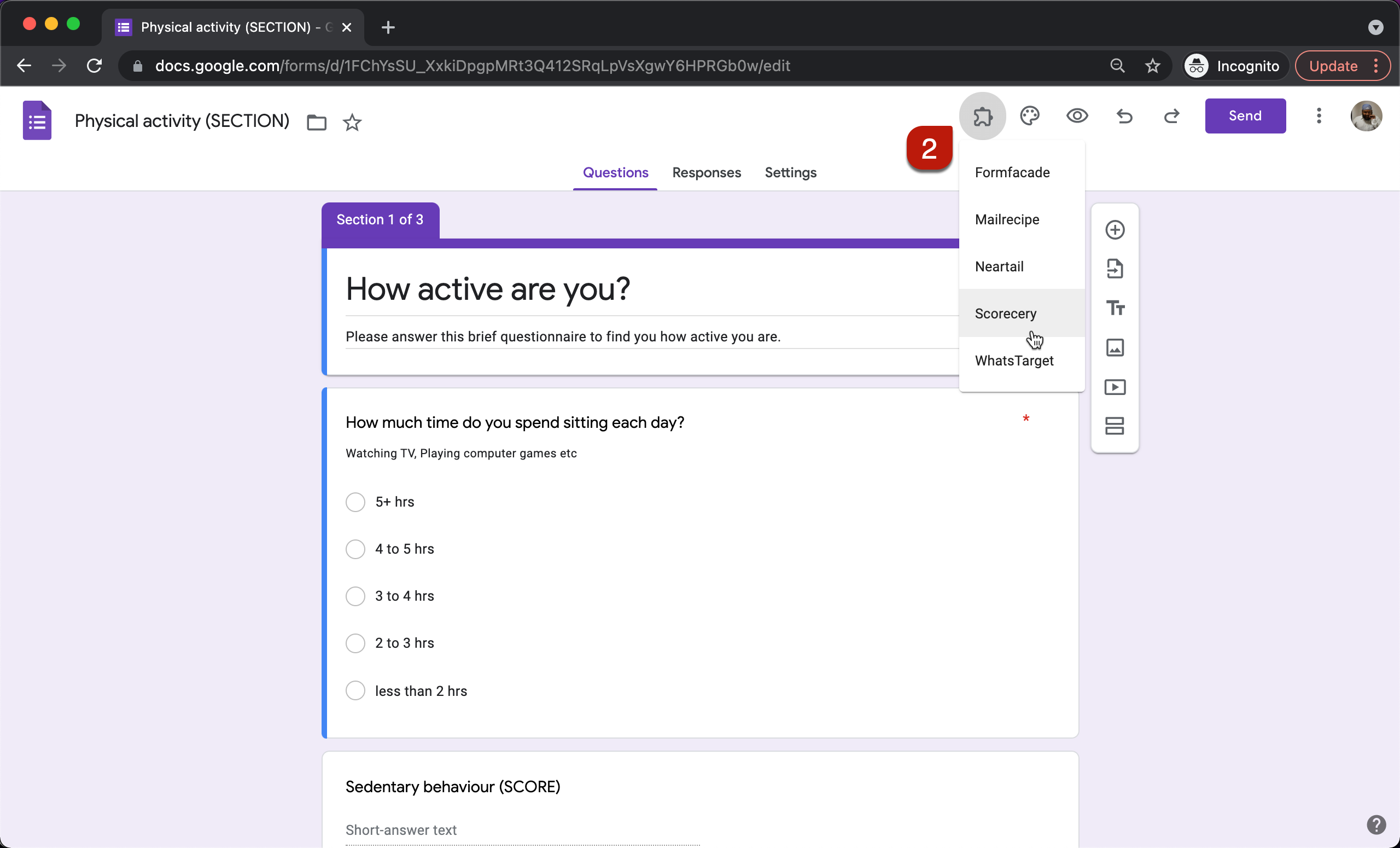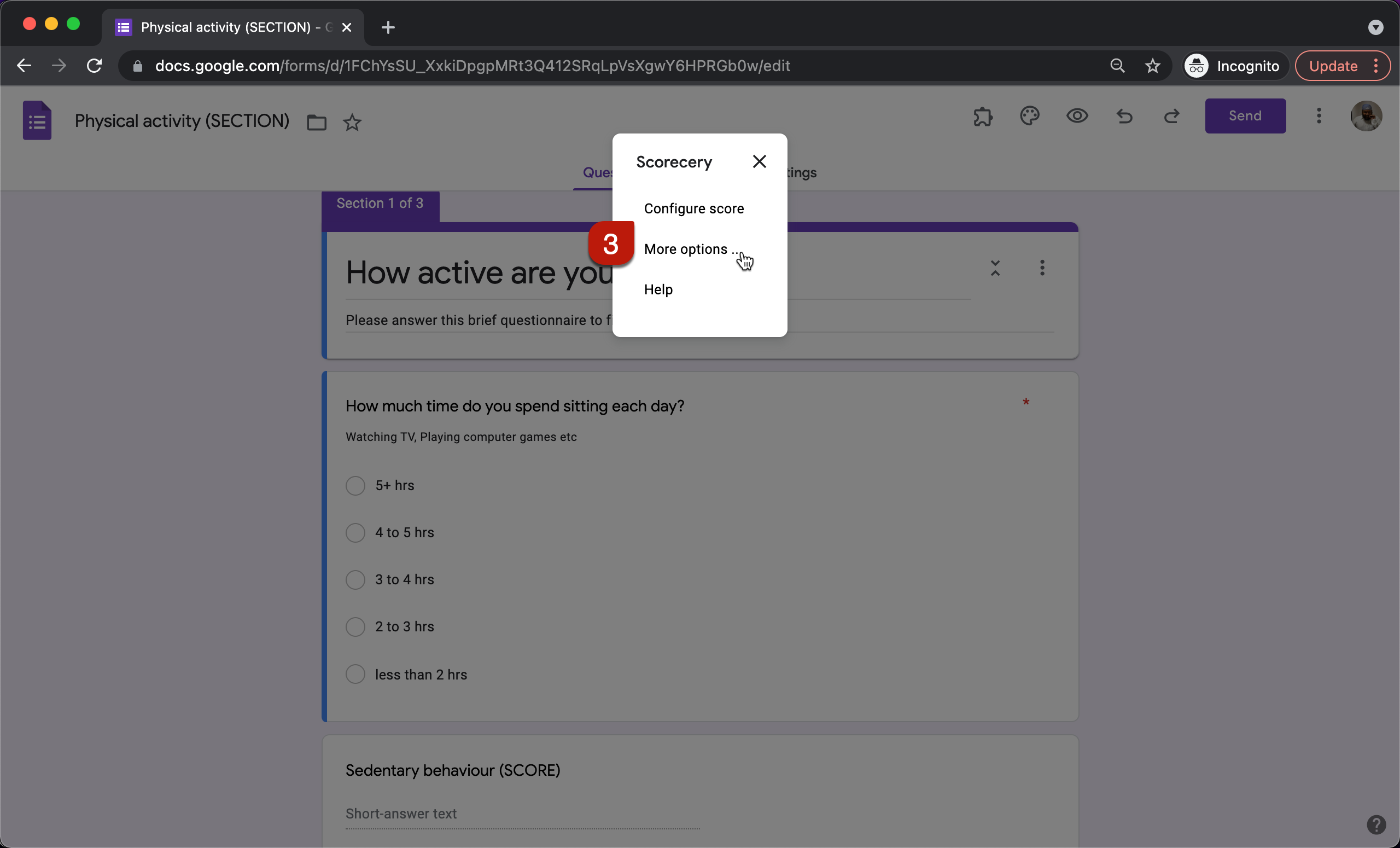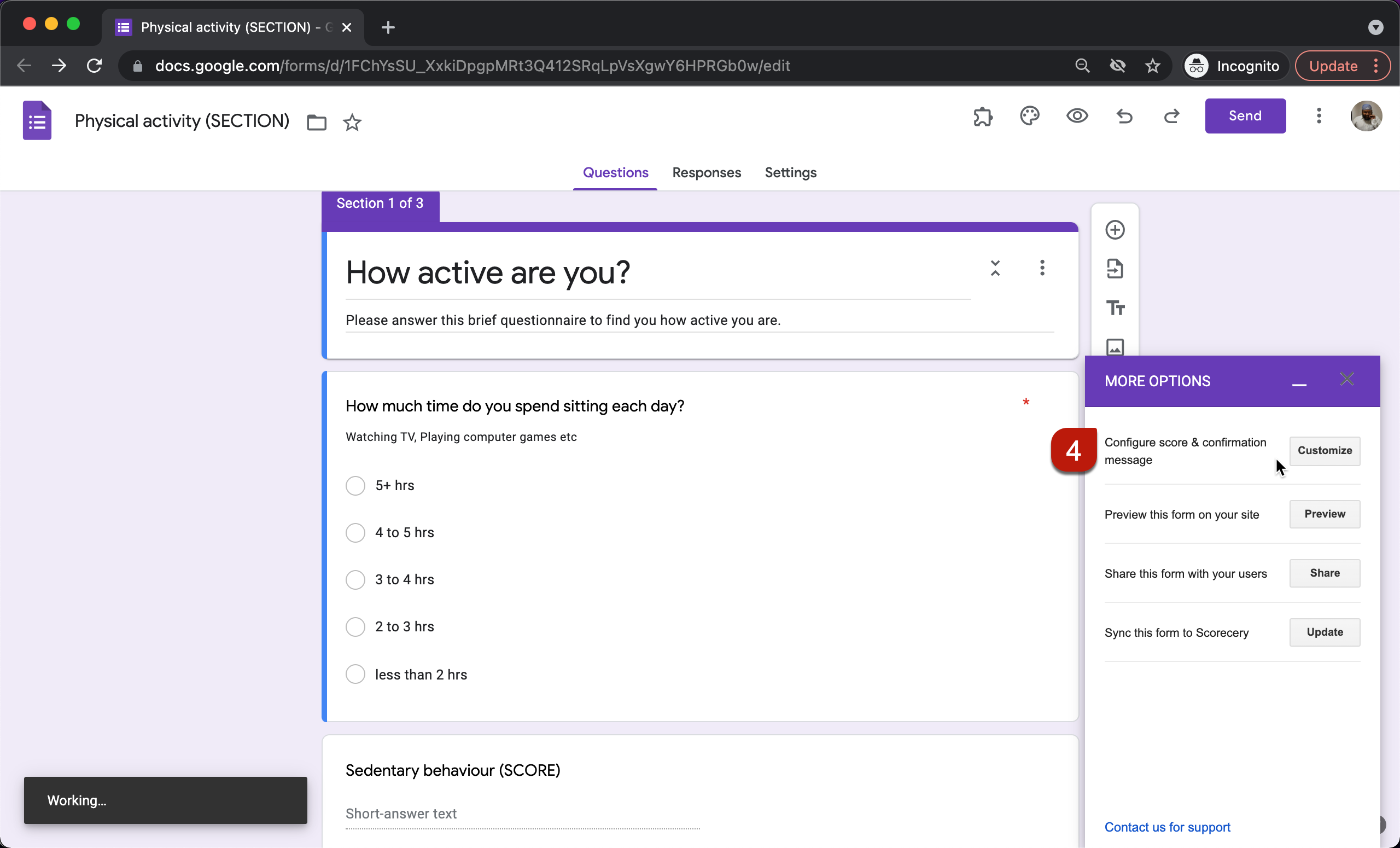#### Assign points

You can assign different points for answer choices in dropdown, multiple choice, checkbox, liner scale & grid questions. You have to repeat steps 5 & 6 below to assign points for all the questions in your form.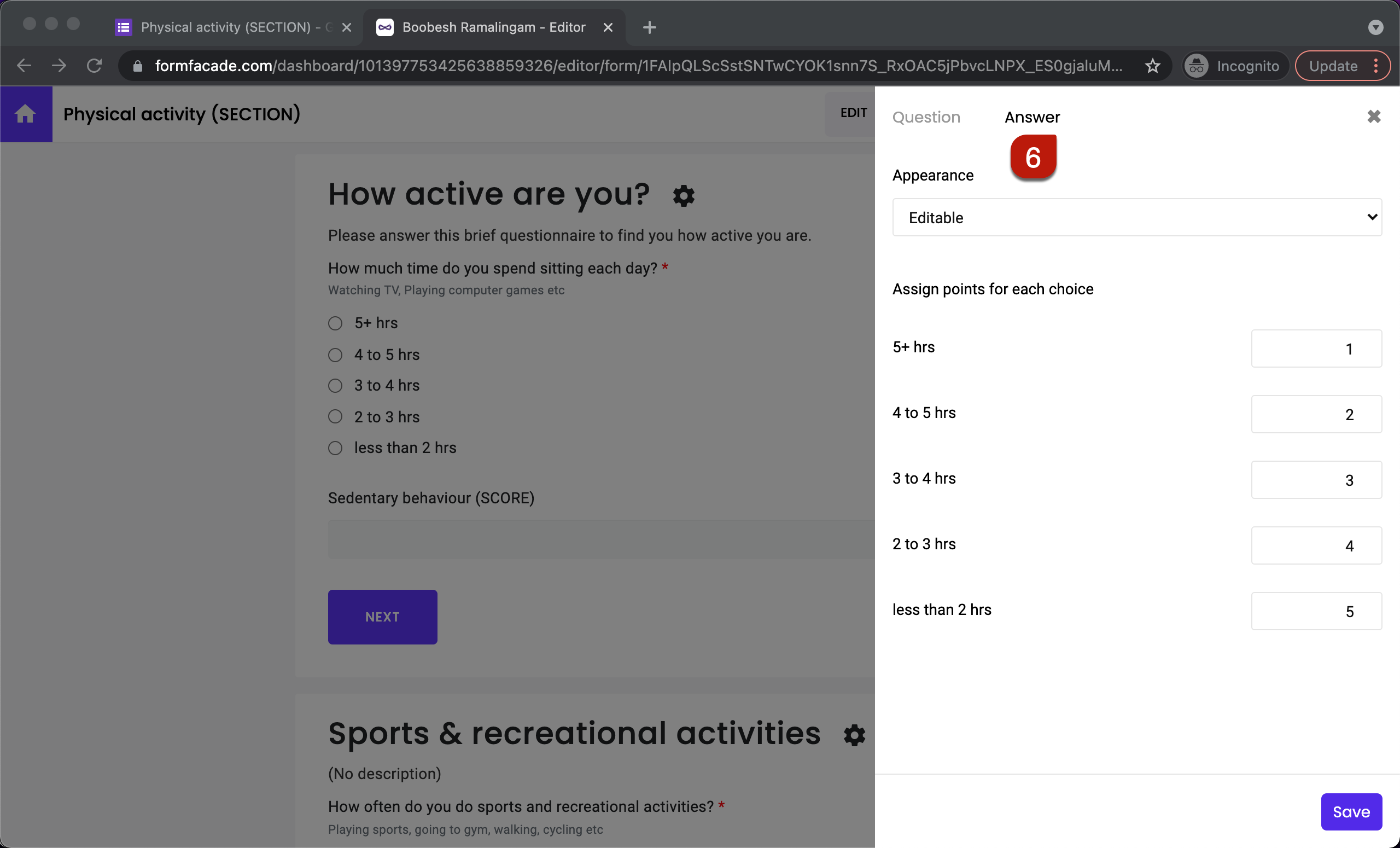#### Calculate section score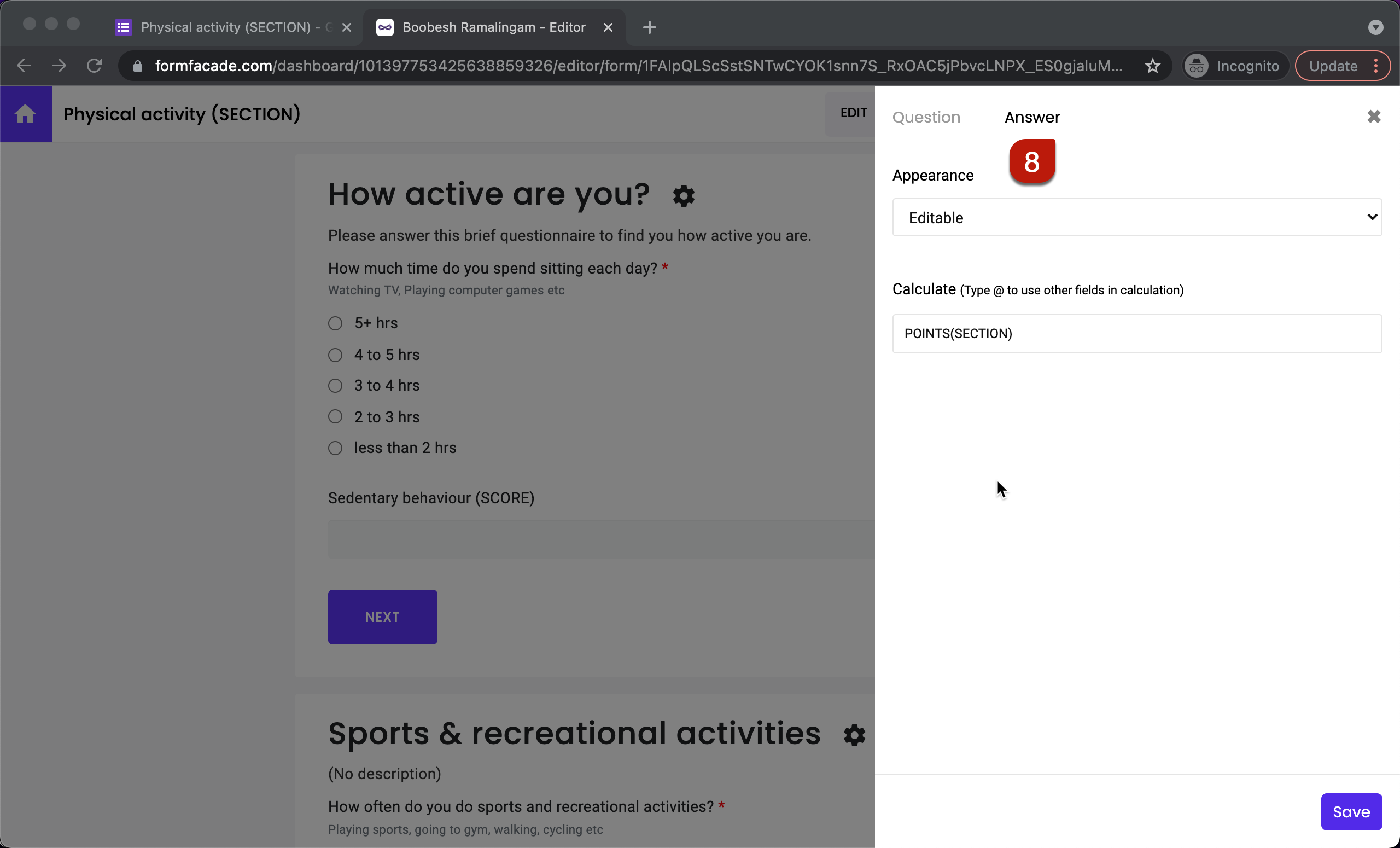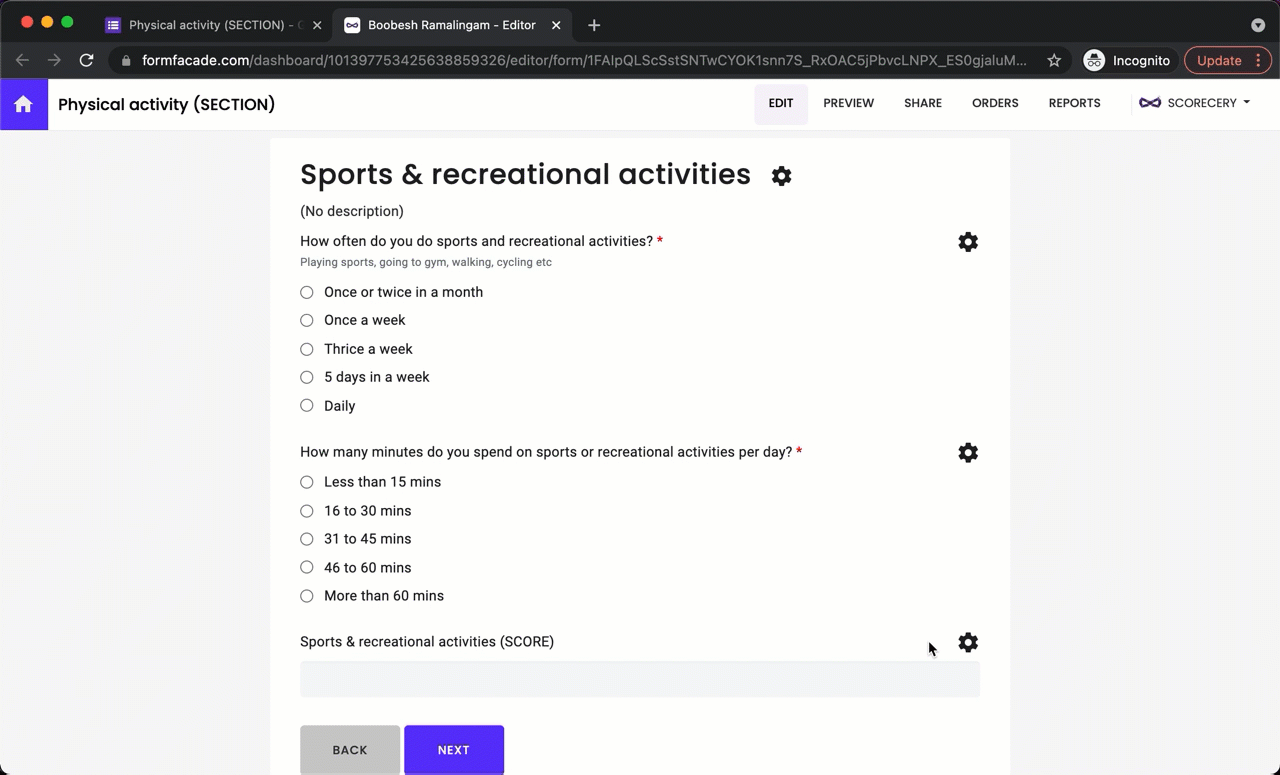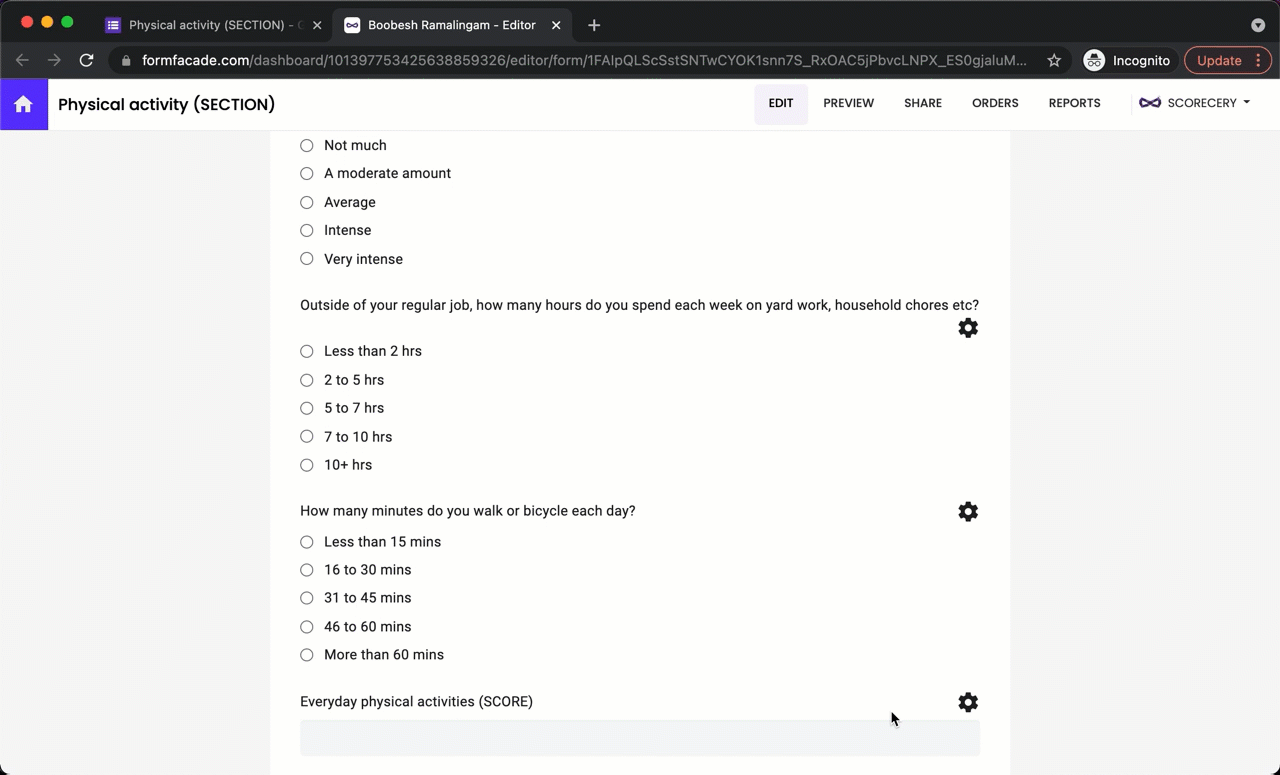#### Overall score

You can use the SUM() formula to add the section scores and calculate the overall score.